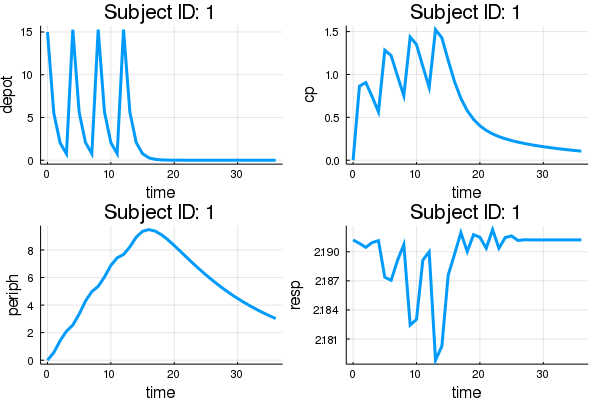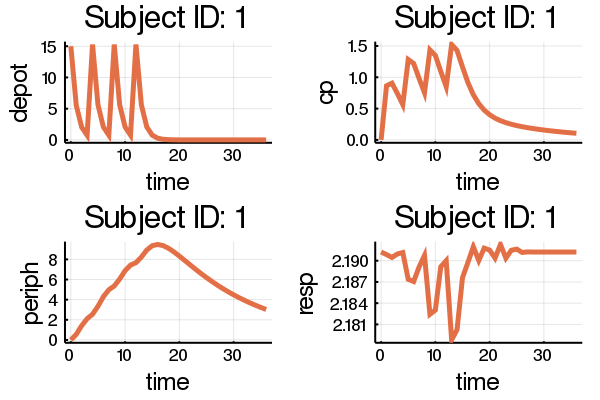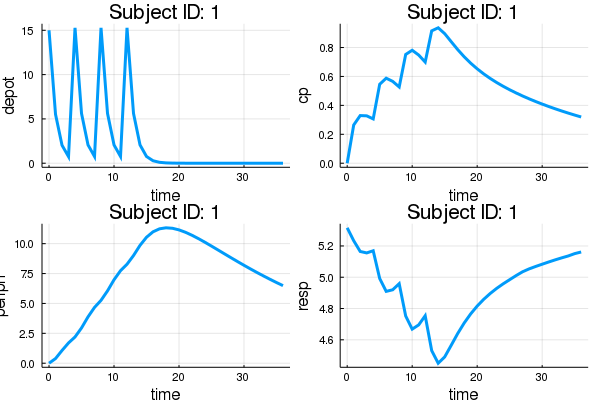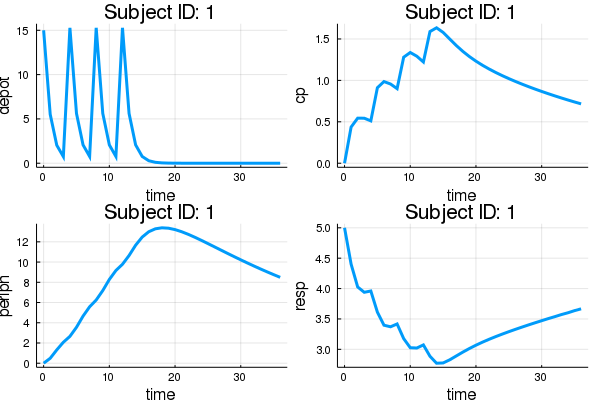Introduction to Pumas

# Introduction to Pumas

This is an introduction to Pumas, a software for pharmaceutical modeling and simulation.

The basic workflow of Pumas is:

1. Build a model.
2. Define subjects or populations to simulate or estimate.
3. Analyze the results with post-processing and plots.

We will show how to build a multiple-response PK/PD model via the `@model` macro, define a subject with multiple doses, and analyze the results of the simulation. This tutorial is made to be a broad overview of the workflow and more in-depth treatment of each section can be found in the subsequent tutorials and documentation.

## Working Example

Let's start by showing a complete simulation code, and then break down how it works.

``````using Pumas, LinearAlgebra

model = @model begin

@param begin
θ ∈ VectorDomain(12)
end

@random begin
η ~ MvNormal(Matrix{Float64}(I, 11, 11))
end

@pre begin
Ka     = θ
CL      = θ*exp(η)
Vc      = θ*exp(η)
Q       = θ*exp(η)
Vp      = θ*exp(η)
Kin     = θ*exp(η)
Kout    = θ*exp(η)
IC50    = θ*exp(η)
IMAX    = θ*exp(η)
γ       = θ*exp(η)
Vmax    = θ*exp(η)
Km      = θ*exp(η)
end

@init begin
Resp = Kin/Kout
end

@dynamics begin
Depot'  = -Ka*Depot
Cent'   =  Ka*Depot - (CL+Vmax/(Km+(Cent/Vc))+Q)*(Cent/Vc)  + Q*(Periph/Vp)
Periph' =  Q*(Cent/Vc)  - Q*(Periph/Vp)
Resp'   =  Kin*(1-(IMAX*(Cent/Vc)^γ/(IC50^γ+(Cent/Vc)^γ)))  - Kout*Resp
end

@derived begin
depot    = Depot
cp     = Cent / Vc
periph = Periph
resp   = Resp
end
end

regimen = DosageRegimen([15,15,15,15], time=[0,4,8,12])
subject = Subject(id=1,evs=regimen)

param = (θ = [
1, # Ka  Absorption rate constant 1 (1/time)
1, # CL   Clearance (volume/time)
20, # Vc   Central volume (volume)
2, # Q    Inter-compartmental clearance (volume/time)
10, # Vp   Peripheral volume of distribution (volume)
10, # Kin  Response in rate constant (1/time)
2, # Kout Response out rate constant (1/time)
2, # IC50 Concentration for 50% of max inhibition (mass/volume)
1, # IMAX Maximum inhibition
1, # γ    Emax model sigmoidicity
0, # Vmax Maximum reaction velocity (mass/time)
2  # Km   Michaelis constant (mass/volume)
],) # single element `NamedTuple`s end with a comma

sim = simobs(model, subject, param)

using Plots
plot(sim)``````In this code, we defined a nonlinear mixed effects model by describing the parameters, the random effects, the dynamical model, and the derived (result) values. Then we generated a subject who receives doses of 15mg every 4 hours, specified parameter values, simulated the model, and generated a plot of the results. Now let's walk through this process!

## Using the Model Macro

First we define the model. The simplest way to do is via the `@model` DSL. Inside of this block we have a few subsections. The first of which is `@param`. In here we define what kind of parameters we have. For this model we will define a vector parameter `θ` of size 12:

``````@param begin
θ ∈ VectorDomain(12)
end``````

Next we define our random effects. The random effects are defined by a distribution from Distributions.jl. For more information on defining distributions, please see the Distributions.jl documentation. For this tutorial, we wish to have a multivariate normal of 11 uncorrelated random effects, so we utilize the syntax:

``````using LinearAlgebra
@random begin
η ~ MvNormal(Matrix{Float64}(I, 11, 11))
end``````

Notice that here we imported `I` from LinearAlgebra and said that our Normal distribution's covariance is said `I`, the identity matrix.

Now we define our pre-processing step in `@pre`. This is where we choose how the parameters, random effects, and the covariates collate. We define the values and give them a name as follows:

``````@pre begin
Ka1     = θ
CL      = θ*exp(η)
Vc      = θ*exp(η)
Q       = θ*exp(η)
Vp      = θ*exp(η)
Kin     = θ*exp(η)
Kout    = θ*exp(η)
IC50    = θ*exp(η)
IMAX    = θ*exp(η)
γ       = θ*exp(η)
Vmax    = θ*exp(η)
Km      = θ*exp(η)
end``````

Next we define the `@init` block which gives the initial values for our differential equations. Any variable not mentioned in this block is assumed to have a zero for its starting value. We wish to only set the starting value for `Resp`, and thus we use:

``````@init begin
Resp = Kin/Kout
end``````

Now we define our dynamics. We do this via the `@dynamics` block. Differential variables are declared by having a line defining their derivative. For our model, we use:

``````@dynamics begin
Depot'  = -Ka*Depot
Cent'   =  Ka*Depot - (CL+Vmax/(Km+(Cent/Vc))+Q)*(Cent/Vc)  + Q*(Periph/Vp)
Periph' =  Q*(Cent/Vc)  - Q*(Periph/Vp)
Resp'   =  Kin*(1-(IMAX*(Cent/Vc)^γ/(IC50^γ+(Cent/Vc)^γ)))  - Kout*Resp
end``````

Lastly we utilize the `@derived` macro to define our post-processing. We can output values using the following:

``````@derived begin
depot  = Depot
cp     = Cent / θ
periph = Periph
resp   = Resp
end``````

## Building a Subject

Now let's build a subject to simulate the model with. A subject defines three components:

1. The dosage regimen
2. The covariates of the individual
3. Observations associated with the individual.

Our model did not make use of covariates so we will ignore (2) for now, and (3) is only necessary for fitting parameters to data which will not be covered in this tutorial. Thus our subject will be defined simply by its dosage regimen.

To do this, we use the `DosageRegimen` constructor. It uses terms from the NMTRAN format to specify its dose schedule. The first value is always the dosing amount. Then there are optional arguments, the most important of which is `time` which specifies the time that the dosing occurs. For example,

``DosageRegimen(15, time=0)``

is a dosage regimen which simply does a single dose at time `t=0` of amount 15. If we use arrays, then the dosage regimen will be the grouping of the values. For example, let's define a dose of amount 15 at times `t=0,4,8`, and `12`:

``regimen = DosageRegimen([15,15,15,15], time=[0,4,8,12])``

Let's define our subject to have `id=1` and this multiple dosing regimen:

``subject = Subject(id=1,evs=regimen)``

## Running a Simulation

The main function for running a simulation is `simobs`. `simobs` on a population simulates all of the population (in parallel), while `simobs` on a subject simulates just that subject. If we wish to change the parameters from the initialized values, then we pass them in. Let's simulate subject 1 with a set of chosen parameters:

``````param = (θ = [
1, # Ka  Absorption rate constant 1 (1/time)
1, # CL   Clearance (volume/time)
20, # Vc   Central volume (volume)
2, # Q    Inter-compartmental clearance (volume/time)
10, # Vp   Peripheral volume of distribution (volume)
10, # Kin  Response in rate constant (1/time)
2, # Kout Response out rate constant (1/time)
2, # IC50 Concentration for 50% of max inhibition (mass/volume)
1, # IMAX Maximum inhibition
1, # γ    Emax model sigmoidicity
0, # Vmax Maximum reaction velocity (mass/time)
2  # Km   Michaelis constant (mass/volume)
],)

sim = simobs(model, subject, param)``````

We can then plot the simulated observations by using the `plot` command:

``````using Plots
plot(sim)``````Note that we can use the attributes from `Plots.jl` to further modify the plot. For example,

``````plot(sim,
color=2,thickness_scaling=1.5,
legend=false, lw=2)``````Notice that in our model we said that there was a single parameter `θ` so our input parameter is a named tuple with just the name `θ`. When we only give the parameters, the random effects are automatically sampled from their distributions. If we wish to prescribe a value for the random effects, we pass initial values similarly:

``````randeffs = (η = rand(11),)
sim = simobs(model, subject, param, randeffs)
plot(sim)``````If a population simulation is required with no random effects, then the values of the η's can be set to zero that will result in a simulation only at the mean level:

``````randeffs = (η = zeros(11),)
sim = simobs(model, subject, param, randeffs)
plot(sim)``````The points which are saved are by default at once every hour until one day after the last event. If you wish to change the saving time points, pass the keyword argument `obstimes`. For example, let's save at every `0.1` hours and run the simulation for 19 hours:

``sim = simobs(model, subject, param, randeffs, obstimes = 0:0.1:19)``

## Handling the SimulatedObservations

The resulting `SimulatedObservations` type has two fields. `sim.times` is an array of time points for which the data was saved. `sim.derived` is the result of the derived variables. From there, the derived variables are accessed by name. For example,

``sim[:cp]``

is the array of `cp` values at the associated time points. We can turn this into a DataFrame via using the `DataFrame` command:

``DataFrame(sim)``

From there, any Julia tools can be used to analyze these arrays and DataFrames. For example, if we wish the plot the result of `depot` over time, we'd use the following:

``plot(sim.times,sim[:depot])``

Using these commands, a Julia program can be written to post-process the program however you like!

## Conclusion

This tutorial covered basic workflow for how to build a model and simulate results from it. The subsequent tutorials will go into more detail in the components, such as:

1. More detailed treatment of specifying populations, dosage regimens, and covariates.
2. Reading in dosage regimens and observations from NMTRAN data.